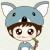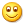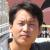## 关于 Java 的自动装箱 (autoboxing) 的一些意想不到的结果

为什么80%的码农都做不了架构师？->>>## 概要

Java 的自动装箱有很多意想不到的结果，其中有些是广为人知的，这些奇怪的结果多数是因为自动装箱对象的缓存导致的。

## == 和 equals 可以也可以不匹配

```All Boolean are cached.
All Byte are cached.
The first auto-boxed char is 0 and the last is 127
The first auto-boxed short is -128 and the last is 127
The first auto-boxed int is -128 and the last is 127
The first auto-boxed long is -128 and the last is 127
No Float are cached.
No Double are cached.```

```All Boolean are cached.
All Byte are cached.
The first auto-boxed char is 0 and the last is 127
The first auto-boxed short is -128 and the last is 127
The first auto-boxed int is -128 and the last is 20000
The first auto-boxed long is -128 and the last is 127
No Float are cached.
No Double are cached.```

## 性能的提升

`The int took 64592 bytes and new ArrayList() with 16000 values took 65048 bytes`

## 自动装箱对象不被垃圾收集

```Map<Long, String> keyValueMap = new WeakHashMap<Long, String>(10000);
for (long i = 1; i <= 8192; i *= 2)
keyValueMap.put(i, "key-" + i);
System.out.println("Before GC: " + keyValueMap);
System.gc();
System.out.println("After GC: " + keyValueMap);```
Map 的键不会被保存，因此你希望用 GC 来清除，但事实并不如你所愿。
```Before GC: {8192=key-8192, 4096=key-4096, 2048=key-2048, 1024=key-1024, 512=key-512, 256=key-256,
128=key-128, 64=key-64, 32=key-32, 16=key-16, 8=key-8, 4=key-4, 2=key-2, 1=key-1}
After GC: {64=key-64, 32=key-32, 16=key-16, 8=key-8, 4=key-4, 2=key-2, 1=key-1}```

## 不要使用自动装箱的对象作为锁

`synchronized("lock one") { // DON'T do this.`

```Object o =
Integer lock = o.hashCode() & 255;
synchronized(lock)  { // DON'T do this either.```

0我想知道为啥不能使用 自动装箱的对象作为锁呢？？？楼主能给个解释吗？
0Integer类默认有个缓冲池，大家看下Integer的嵌套类IntegerCache就清楚了。

## 1. == 和 equals 可以也可以不匹配

== 比较的是内存地址，是同一个对象则返回true。 这和一般的对象比较没有区别。

## 3. 自动装箱对象不被垃圾收集

请注意，只是缓存起来的没被收集，这是单例的特性。

## 4. 不要使用自动装箱的对象作为锁

和使用string对象一样，容易造成死锁。

private static class IntegerCache {
static final int low = -128;
static final int high;
static final Integer cache[];
static {
// high value may be configured by property
int h = 127;
String integerCacheHighPropValue =
sun.misc.VM.getSavedProperty("java.lang.Integer.IntegerCache.high");
if (integerCacheHighPropValue != null) {
int i = parseInt(integerCacheHighPropValue);
i = Math.max(i, 127);
// Maximum array size is Integer.MAX_VALUE
h = Math.min(i, Integer.MAX_VALUE - (-low));
}

high = h;
cache = new Integer[(high - low) + 1];
int j = low;
for(int k = 0; k < cache.length; k++)
cache[k] = new Integer(j++);
}
private IntegerCache() {}
}
01.  两个操作数都是包装类型时进行的是引用比较即==

```Integer a = new Integer(100);
Integer b = new Integer(100);
a == b ; //false;```

相反：

```Integer a = Ingeter.valueOf(100);
Integer a = Ingeter.valueOf(100);
a == b ; // true;```

原因是Integer.valueOf()会取缓冲池里的对象加以利用。

这也是我们提倡使用Integer.valueOf()而不是new Integer()的原因，就像我们不提倡new  String()一样。

2.  一方是原始类型，一方是包装类时，进行拆箱，即值比较。

`100 == new Integer(100) ; // tue;`

0
g